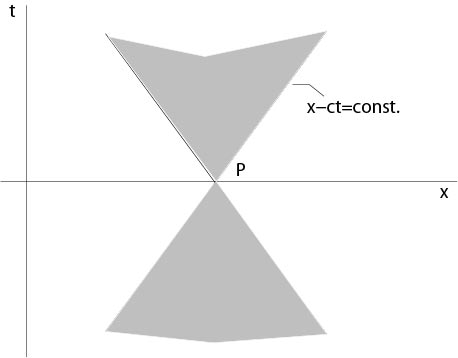$$\newcommand{\id}{\mathrm{id}}$$ $$\newcommand{\Span}{\mathrm{span}}$$ $$\newcommand{\kernel}{\mathrm{null}\,}$$ $$\newcommand{\range}{\mathrm{range}\,}$$ $$\newcommand{\RealPart}{\mathrm{Re}}$$ $$\newcommand{\ImaginaryPart}{\mathrm{Im}}$$ $$\newcommand{\Argument}{\mathrm{Arg}}$$ $$\newcommand{\norm}{\| #1 \|}$$ $$\newcommand{\inner}{\langle #1, #2 \rangle}$$ $$\newcommand{\Span}{\mathrm{span}}$$

# 4.1: One-Dimensional Wave Equation

$$\newcommand{\vecs}{\overset { \rightharpoonup} {\mathbf{#1}} }$$ $$\newcommand{\vecd}{\overset{-\!-\!\rightharpoonup}{\vphantom{a}\smash {#1}}}$$$$\newcommand{\id}{\mathrm{id}}$$ $$\newcommand{\Span}{\mathrm{span}}$$ $$\newcommand{\kernel}{\mathrm{null}\,}$$ $$\newcommand{\range}{\mathrm{range}\,}$$ $$\newcommand{\RealPart}{\mathrm{Re}}$$ $$\newcommand{\ImaginaryPart}{\mathrm{Im}}$$ $$\newcommand{\Argument}{\mathrm{Arg}}$$ $$\newcommand{\norm}{\| #1 \|}$$ $$\newcommand{\inner}{\langle #1, #2 \rangle}$$ $$\newcommand{\Span}{\mathrm{span}}$$ $$\newcommand{\id}{\mathrm{id}}$$ $$\newcommand{\Span}{\mathrm{span}}$$ $$\newcommand{\kernel}{\mathrm{null}\,}$$ $$\newcommand{\range}{\mathrm{range}\,}$$ $$\newcommand{\RealPart}{\mathrm{Re}}$$ $$\newcommand{\ImaginaryPart}{\mathrm{Im}}$$ $$\newcommand{\Argument}{\mathrm{Arg}}$$ $$\newcommand{\norm}{\| #1 \|}$$ $$\newcommand{\inner}{\langle #1, #2 \rangle}$$ $$\newcommand{\Span}{\mathrm{span}}$$

The one-dimensional wave equation is given by

\begin{equation}
\label{waveone}
\dfrac{1}{c^2}u_{tt}-u_{xx}=0,
\end{equation}
where $$u=u(x,t)$$ is a scalar function of two variables and $$c$$ is a positive constant. According to previous considerations, all $$C^2$$-solutions of the wave equation are

\begin{equation}
\label{wavegen}
u(x,t)=f(x+ct)+g(x-ct),
\end{equation}

with arbitrary $$C^2$$-functions $$f$$ and $$g$$

The Cauchy initial value problem for the wave equation is to find a $$C^2$$-solution of

\begin{eqnarray*}
\dfrac{1}{c^2}u_{tt}-u_{xx}&=&0\\
u(x,0)&=&\alpha(x)\\
u_t(x,0)&=&\beta(x),
\end{eqnarray*}

where $$\alpha,\ \beta\in C^2(-\infty,\infty)$$ are given.

Theorem 4.1. There exists a unique $$C^2(\mathbb{R}^1\times\mathbb{R}^1)$$-solution of the Cauchy initial value problem, and this solution is given by d'Alembert's1 formula

\begin{equation}
\label{waveform}
u(x,t)=\dfrac{\alpha(x+ct)+\alpha(x-ct)}{2}+\dfrac{1}{2c}\int_{x-ct}^{x+ct}\ \beta(s)\ ds.
\end{equation}

Proof. Assume there is a solution $$u(x,t)$$ of the Cauchy initial value problem, then it follows from (\ref{wavegen}) that

\begin{eqnarray}
\label{ini1}
u(x,0)&=&f(x)+g(x)=\alpha(x)\\
\label{ini2}
u_t(x,0)&=&cf'(x)-cg'(x)=\beta(x).
\end{eqnarray}

From (\ref{ini1}) we obtain

$f'(x)+g'(x)=\alpha'(x),$

which implies, together with (\ref{ini2}), that

$\begin{eqnarray*} \label{12a} f'(x)&=&\dfrac{\alpha'(x)+\beta(x)/c}{2}\\ \label{12b}g'(x)&=&\dfrac{\alpha'(x)-\beta(x)/c}{2}. \end{eqnarray*}$

Then

$\begin{eqnarray*} f(x)&=&\dfrac{\alpha(x)}{2}+\dfrac{1}{2c}\int_0^x\ \beta(s)\ ds +C_1\\ g(x)&=&\dfrac{\alpha(x)}{2}-\dfrac{1}{2c}\int_0^x\ \beta(s)\ ds +C_2. \end{eqnarray*}$

The constants $$C_1$$, $$C_2$$ satisfy

$C_1+C_2=f(x)+g(x)-\alpha(x)=0,$

see (\ref{ini1}). Thus each $$C^2$$-solution of the Cauchy initial value problem is given by d'Alembert's formula. On the other hand, the function $$u(x,t)$$ defined by the right hand side of (\ref{waveform}) is a solution of the initial value problem.

$$\Box$$

Corollaries. 1. The solution $$u(x,t)$$ of the initial value problem depends on the values of $$\alpha$$ at the endpoints of the interval $$[x-ct,x+ct]$$ and on the values of $$\beta$$ on this interval only, see Figure 4.1.1. The interval $$[x-ct,x+ct]$$ is called {\it domain of dependence}.Figure 4.1.1: Interval of dependence

2. Let $$P$$ be a point on the $$x$$-axis. Then we ask which points $$(x,t)$$ need values of $$\alpha$$ or $$\beta$$ at $$P$$ in order to calculate $$u(x,t)$$? From the d'Alembert formula it follows that this domain is a cone, see Figure 4.2.1. This set is called domain of influence.Figure 4.2.1: Domain of influence

1 d'Alembert, Jean Babtiste le Rond, 1717-1783

## Contributors

• Integrated by Justin Marshall.